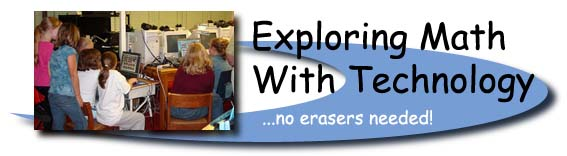Wicomico County Professional Day, January 30, 2004
Intermediate Session

 Software Applications on our Network:

1. Kidspiration Activities Math Standards: Capacity (Gr. 4) 3C.2.c; (Gr. 5) 3C.2.b; Polygons (Gr. 3, 5) 2A.1.b; (Gr. 4) 2A.2.a Area and perimeter (Gr. 3, 4, 5) 3C.1.a; (Gr. 3, 4, 5) 3C.1.b
2. "Red Pockets" and US money: Grade 5 students write to friends in Taiwan about our currency.
3. See what's happening in the computer lab - Math Standard: (Gr. 5) 6C.1.e; (Gr. 3) 6C.1.d - Two Excel spreadsheet activities. Students were given a certain amount of money to "spend" on items. Also includes examples of pictographs, bar and line graphs made with Graph Club. Math Standard: (Gr. 3) 4A.1.d
 Online Interactive Math Websites:

Page numbers for BCRs refer to pages in the handouts for this session.

Standard 3.0 - Knowledge of Measurement

1. Teaching Time - Math Standard: (Gr. 3) 3A.1.b; (Gr. 4) 3C.1.c; (Gr. 5) 3C.2.a
2. Elapsed Time - Math Standard: (Gr. 4) 3C.1.c; ( Gr. 5) 3C.2.a Sample BCR (p. 1)
3. Measure Angles - Math Standard (Gr. 5) 3B.2.a
4. Perimeter - Math Standard: (Gr. 3, 4, 5) 3C.1.a
5. Area Explorer - irregular shapes - Math Standard: (Gr. 3, 4, 5) 3C.1.b Sample BCR (p. 2) and Sample BCR (p. 3)
6. Find the area of a rectangle - centimeters, Math Standard: (Gr. 3, 4, 5) 3C.1.b
7. Thermometer - [How to use] - Math Standard: (Gr. 3) 3A.1.c
8. Measuring Cylinder [How to use] - Math Standard: (Gr. 3) 3B.1.b; (Gr. 4, 5) 3B.1.a
9. Weight - to the nearest gram, Math Standard: (Gr. 3) 3A.1.d; (Gr. 4) 3A.1.b (Gr. 5) 3A.1.a Sample BCR (p. 4)
10. Measuring Scale [How to use] - Math Standard: (Gr. 3) 3A.1.d; (Gr. 4) 3A.1.b; (Gr. 5) 3A.1.a
11. Rulers - Sample BCR (p. 5)
1. Ruler - [How to use] - Math Standard: (Gr. 3, 4, 5) 3B.1.a;
2. Ruler centimeters and millimeters - Math Standard: (Gr. 3, 4, 5) 3B.1.a Sample BCR (p.6)
3. Measuring lines centimeters - Math Standard: (Gr. 3, 4, 5) 3B.1.a

Standard 1.0 - Knowledge of Algebra, Patterns, or Functions

1. Number chart up to 1000 and calculator. - Math Standard: (Gr. 3, 4) 1A.1.a
2. Whole Number Cruncher - Function Machine (*,+) - Math Standard: (Gr. 3) 1A.1.d; (Gr. 4, 5) 1A.1.c; Sample BCR (p. 7)
3. Function Machine - (+,-,*,/ - allows 2 step operations) Math Standard: (Gr. 3) 1A.1.d; (Gr. 4, 5) 1A.1.c; Sample BCR (p. 8)
4. Number Lines - Math Standard: (Gr. 3, 4) 1A.1.a (Gr. 5) 6A.1.e Sample BCR (p. 9)
5. Virtual Counting Stick - Math Standard: (Gr. 3, 4) 1A.1.a (Gr. 5) 6A.1.e
6. Identifying fractions on a number line - Math Standard: (Gr. 3) 1C.1.b; (Gr. 4) 1C.1.a Sample BCR (p. 10)
7. Mixed Numbers on number lines Math Standard: (Gr. 4, 5) 1.C.1.a Sample BCR (p. 11)
8. Coordinates and (simplified) Coordinates and plot the points - Math Standard: (Gr. 4) 1C.1.b; (Gr. 5) 1.C.1 Sample BCR (p. 12)
9. Equivalence - Math Standard: (Gr. 3, 4, 5) 1B.1.a Sample BCR (p. 13) Sample #2 BCR (p. 14)
10. Equivalence - Math Standard: (Gr. 3, 4, 5) 1B.1.a Balance equations using +,-,*, and / Sample BCR (p. 15)
Standard 2.0 - Knowledge of Geometry
1. Symmetry with Pattern Blocks - Math Standard: (Gr. 3) 2E.2 Sample BCR (p. 16)
2. Polygon Playground - Math Standard: (Gr. 3, 5) 2A.1.b; (Gr. 4) 2A.2.a (Gr. 3, 4, 5) 2D.1.a
3. Congruent Triangles - Math Standard: (Gr. 3, 4, 5) 2D.1.a Sample BCR (p. 17)
4. Exploring Geometric Solids - Math Standard: (Gr. 3, 5) 2B.1.a; (Gr. 4) 2B.1.b
5. Geoboard - Perimeter and Area; NCTM Geoboard (Gr. 3, 4, 5) 3C.1.a; Geometric Shapes (Gr. 3, 5) 2A.1.b; Angles (Gr. 3, 4,5) 2A.2.a; Geoboard Activities
6. Exploring properties of parallelograms and rectangles - Math Standard: (Gr. 3) 2A.1.c; (Gr. 4, 5) 2A.2.a
7. Translation, reflection and rotation - Math Standard: (Gr. 3, 4, 5) 2E.1.a Transformation Golf
1. Flips, slides and turns (submitted by Julie Owens)
2. Rotations - Sample BCR (p. 18)
3. Reflections
4. Translations

Standard 6.0 - Knowledge of Number Relationships or Computation

1. Mixed Numbers With Circles - Math Standard: (Gr. 4) 6A.2.e; (Gr. 5) 6A.1.a
2. Pattern Blocks Math Standard: (Gr. 3, 4) 6A.2.a; (Gr. 5) 6A.1.a; lesson plans by Cynthia Lanius - Pattern Blocks and Fractions, Sample BCR (p. 19)
3. Visualizing Fractions - Math Standard: (Gr. 3, 4) 6A.2.a; (Gr. 5) 6A.1.a
4. Fractions, decimals, ratios, percents [How to use] - Math Standard: (Gr. 4) 6A.2.c; (Gr. 5) 6A.1.c;
5. Number line with decimals - Math Standard: (Gr. 4) 6A.2.c; (Gr. 5) 6A.1.b - Shows the decimal on a number line. Sample BCR (p. 20)
6. The Decifractator Fractions and equivalent decimals - Math Standard: (Gr. 5) 6A.1.c
7. Greatest Common Factor and Least Common Multiple - Math Standard: GCF (Gr. 5) 6B.1.c; LCM (Gr. 5) 6B.1.d
8. Number chart up to 1000 and calculator (for GCF) - Math Standard: (Gr. 5) 6B.1.c
9. Tables Tester - Math Standard (Gr. 3) 6C.1.f; (Gr. 4) 6C.1.c; (Gr. 5) 6C.1.a Sample BCR (p. 21)

Standard 5.0 - Knowledge of Probability

1. Probability and Basic spinner - Math Standard: (Gr. 3,4,5) 5B.1.a Sample BCR (p. 22)
2. Probability - More advanced spinner, explanations and discussion questions; Math Standard: (Gr. 3,4,5) 5B.1.a

Standard 4.0 - Knowledge of Statistics

1. Stem and Leaf Plotter - Math Standard: (Gr. 5) 4A.1.b
2. Line Plots - Gathering data and making a line plot, Math Standard: (Gr. 3) 4A.1.e; (Gr. 4) 4A.1.b Sample BCR (p. 23)
 Online Global Projects at Delmar:
1. Comparing the cost of stamps in the USA and Taiwan (Gen Y students in grade 5)
2. Mystery Number Trains Math Standard: (Gr. 3, 4, 5) 1B.1.a Using Online Integer Bars
3. Mrs. Carrie Lewis' grade 3 students compare "pocket money" with friends in Japan.